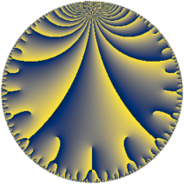# Properties

 Label 75.9.cLevel $75$ Weight $9$ Character orbit 75.c Rep. character $\chi_{75}(26,\cdot)$ Character field $\Q$ Dimension $48$ Newform subspaces $8$ Sturm bound $90$ Trace bound $3$

# Related objects

## Defining parameters

 Level: $$N$$ $$=$$ $$75 = 3 \cdot 5^{2}$$ Weight: $$k$$ $$=$$ $$9$$ Character orbit: $$[\chi]$$ $$=$$ 75.c (of order $$2$$ and degree $$1$$) Character conductor: $$\operatorname{cond}(\chi)$$ $$=$$ $$3$$ Character field: $$\Q$$ Newform subspaces: $$8$$ Sturm bound: $$90$$ Trace bound: $$3$$ Distinguishing $$T_p$$: $$2$$, $$7$$

## Dimensions

The following table gives the dimensions of various subspaces of $$M_{9}(75, [\chi])$$.

Total New Old
Modular forms 86 54 32
Cusp forms 74 48 26
Eisenstein series 12 6 6

## Trace form

 $$48 q + 22 q^{3} - 5648 q^{4} + 1998 q^{6} - 3656 q^{7} - 690 q^{9} + O(q^{10})$$ $$48 q + 22 q^{3} - 5648 q^{4} + 1998 q^{6} - 3656 q^{7} - 690 q^{9} + 26132 q^{12} + 4004 q^{13} + 633224 q^{16} + 147640 q^{18} - 33770 q^{19} + 182658 q^{21} - 817460 q^{22} - 1432386 q^{24} + 1377982 q^{27} + 2472724 q^{28} - 13374 q^{31} - 2203900 q^{33} + 7423684 q^{34} + 3058266 q^{36} - 7342956 q^{37} - 5520522 q^{39} + 10684860 q^{42} - 679036 q^{43} - 13466344 q^{46} - 16336588 q^{48} + 41536858 q^{49} - 4715922 q^{51} - 8301936 q^{52} + 14114292 q^{54} + 1151884 q^{57} + 25045340 q^{58} - 36959094 q^{61} - 17619096 q^{63} - 52712584 q^{64} + 90514230 q^{66} + 42108244 q^{67} + 73955532 q^{69} - 56352840 q^{72} + 74619344 q^{73} - 34760548 q^{76} - 138195880 q^{78} - 185493440 q^{79} + 161445198 q^{81} - 118669240 q^{82} - 80905392 q^{84} + 106239820 q^{87} + 487275540 q^{88} - 442822454 q^{91} - 431779356 q^{93} - 13838696 q^{94} + 781827654 q^{96} - 35716296 q^{97} - 74078190 q^{99} + O(q^{100})$$

## Decomposition of $$S_{9}^{\mathrm{new}}(75, [\chi])$$ into newform subspaces

Label Dim $A$ Field CM Traces $q$-expansion
$a_{2}$ $a_{3}$ $a_{5}$ $a_{7}$
75.9.c.a $1$ $30.553$ $$\Q$$ $$\Q(\sqrt{-3})$$ $$0$$ $$-81$$ $$0$$ $$4273$$ $$q-3^{4}q^{3}+2^{8}q^{4}+4273q^{7}+3^{8}q^{9}+\cdots$$
75.9.c.b $1$ $30.553$ $$\Q$$ $$\Q(\sqrt{-3})$$ $$0$$ $$81$$ $$0$$ $$-4273$$ $$q+3^{4}q^{3}+2^{8}q^{4}-4273q^{7}+3^{8}q^{9}+\cdots$$
75.9.c.c $2$ $30.553$ $$\Q(\sqrt{-14})$$ None $$0$$ $$-90$$ $$0$$ $$3500$$ $$q+\beta q^{2}+(-45-3\beta )q^{3}-248q^{4}+\cdots$$
75.9.c.d $2$ $30.553$ $$\Q(\sqrt{-1})$$ $$\Q(\sqrt{-15})$$ $$0$$ $$0$$ $$0$$ $$0$$ $$q+17iq^{2}-3^{4}iq^{3}-33q^{4}+1377q^{6}+\cdots$$
75.9.c.e $10$ $30.553$ $$\mathbb{Q}[x]/(x^{10} + \cdots)$$ None $$0$$ $$-25$$ $$0$$ $$1960$$ $$q+\beta _{2}q^{2}+(-2-\beta _{2}+\beta _{4})q^{3}+(-155+\cdots)q^{4}+\cdots$$
75.9.c.f $10$ $30.553$ $$\mathbb{Q}[x]/(x^{10} + \cdots)$$ None $$0$$ $$25$$ $$0$$ $$-1960$$ $$q+\beta _{2}q^{2}+(2-\beta _{2}-\beta _{4})q^{3}+(-155+\cdots)q^{4}+\cdots$$
75.9.c.g $10$ $30.553$ $$\mathbb{Q}[x]/(x^{10} - \cdots)$$ None $$0$$ $$112$$ $$0$$ $$-7156$$ $$q+\beta _{1}q^{2}+(11+2\beta _{1}+\beta _{2})q^{3}+(-79+\cdots)q^{4}+\cdots$$
75.9.c.h $12$ $30.553$ $$\mathbb{Q}[x]/(x^{12} + \cdots)$$ None $$0$$ $$0$$ $$0$$ $$0$$ $$q-\beta _{3}q^{2}+(\beta _{2}-\beta _{3})q^{3}+(-142-\beta _{5}+\cdots)q^{4}+\cdots$$

## Decomposition of $$S_{9}^{\mathrm{old}}(75, [\chi])$$ into lower level spaces

$$S_{9}^{\mathrm{old}}(75, [\chi]) \cong$$ $$S_{9}^{\mathrm{new}}(3, [\chi])$$$$^{\oplus 3}$$$$\oplus$$$$S_{9}^{\mathrm{new}}(15, [\chi])$$$$^{\oplus 2}$$